### Seeing is believing!

Before you order, simply sign up for a free user account and in seconds you'll be experiencing the best in CFA exam preparation.

### Subject 3. Interest rate swaps

Example:

On December 15 XYZ enters into \$50 million NP swap with ABSwaps. Payments will be on 15th of March, June, September, December for one year, based on LIBOR. XYZ will pay 7.5% fixed and ABSwaps will pay LIBOR. Interest based on exact day count and 360 days (30 per month). In general the cash flow to the fixed payer will be

Notional principal x (LIBOR - Fixed rate) x (Days / 360 or 365)

• The payments in this swap are: 50,000,000 x (LIBOR - 0.075) x (days/360). Note that payments are netted.
• We denote Li(m) as the m-day LIBOR on day i. If i = 1, we are referring to 90 days after day 0 in this example, and L1(90) is the 90-day LIBOR on day 90.
• q represents the factor (days/360).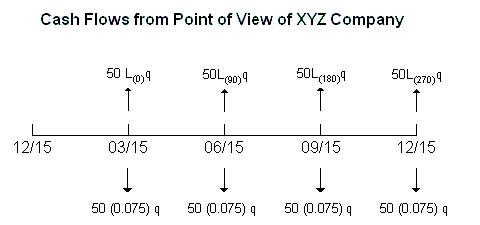After-the-Fact Payments in Plain Vanilla Interest Rate Swap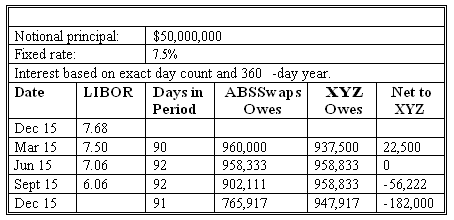Note: This combination of LIBORS on the above dates represents only one of an infinite number of possible outcomes to the swap. They are used to illustrate how the payments are determined and not the likely results.

How is the fixed rate determined?

Remember that swap pricing means to determine the fixed rate and any relevant terms at the start of the swap.

A digression on floating-rate securities: We denote B0(ti) as the present value factor on a zero-coupon instrument paying \$1 at its maturity date. The price of a LIBOR zero coupon bond for maturity of ti days is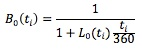As an example, to discount payments 180 days later, we multiply the payment amount by the following factor: B0(180) = 1 / {1 + [L0(180) x (180/360)]}. We can think of this discount factor as the value of spot LIBOR deposit that pays \$1 180 days later.

On day 0, the floating rate is set for the first period and the interest to be paid at that rate on day 90. Then on day 90, the rate is set for the second period and the interest is paid on day 180. This process continues so that on day 270 the rate is set for the last period, and the final interest payment and the principal are paid on day 360. Let the principal be 1.0.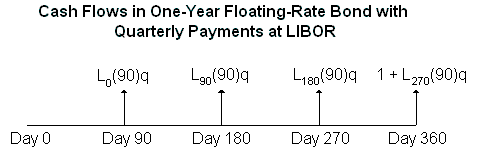Starting at the maturity date and working back, we can see that the value is par at the start and on each coupon reset date.

• Suppose today is day 270, and the LIBOR on that day is L270(90). Remember that this rate is the 90-day LIBOR in the market at the time. Looking ahead to day 360, we anticipate receiving 1.0, the final principal payment, plus L270(90) x 90/360. What is the value of this amount on day 270? We would discount it by the appropriate 90-day LIBOR in the following manner:

Value on day 270 = (Payment on day 360) x (One-period discount factor) = [1.0 + L270(90) x (90/360)] x {1 / [1 + (L270(90) x (90/360))]} = 1.0.

• We continue this procedure, stepping back until we reach time 0.

Payments in an interest rate swap do not include an initial and final exchange of notional principals. If we introduce the notional principal payments as though they were actually made, we have not done any harm. The cash flows on the swap are still the same. However, by adding the notional principals, we can separate the cash flow streams of an interest rate swap into those of a fixed-rate bond and a floating-rate bond.

The value of a fixed-rate bond (q = days/360, and R is the fixed rate):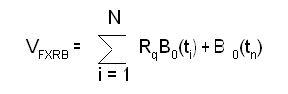Here the summation simply represents the sum of the present value factors for each payment. The expression B0(tn) is the present value factor for the final hypothetical notional principal payment of 1.0.

The value of a floating-rate bond:VFLRB = 1 (at time 0 or a payment date).
The value of the swap (pay fixed, receive floating) is, therefore, VS = VFLRB - VFXRB.

To price the swap at the start, set this value to zero and solve for R:In the above example, suppose the term structure of LIBOR at the start of the contract is: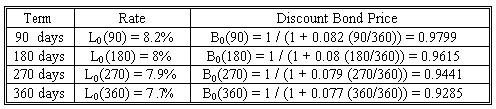With q = 90/360, then 1/q = 360/90 and the fixed rate would, therefore, be R = (360/90) [(1 - 0.9285) / (0.9799 + 0.9615 + 0.9441 + 0.9285)] = 0.075.

Thus, the rate would be 7.5%.

See basic questions for other examples.

#### Practice Question 1

Quantum Electronics enters into a two-year \$20 million notional principal interest rate swap in which it promises to pay a fixed rate and receive payments at LIBOR. The payments are made every six months based on the assumption of 30 days per month and 360 days in a year. The term structure of LIBOR interest rates is given as follows: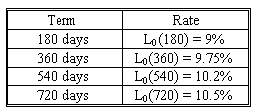What should the fixed rate be?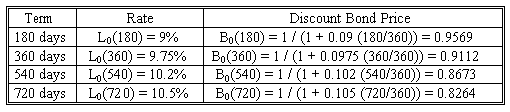With q = 180/360, then 1/q = 360/180 and the fixed rate would, therefore, be R = (360/180) [(1 - 0.8264) / (0.9569 + 0.9112 + 0.8673 + 0.8264)] = 0.0975.

Thus, the rate would be 9.75%. The swap fixed payments would be \$20,000,000 x 0.0975 x 180/360 = \$975,000.

#### Practice Question 2

When the Quantum Electronic swap was first established, the first floating payment was set at the 180-day rate of 9%. For a \$1 notional principal, the payment would be 0.09 x (180/360) = 0.045. The fixed payment is at 9.75%, so it would be 0.0975 (180/360) = 0.04875. To value the swap 90 days into its life, we need the new term structure of interest rates as follows: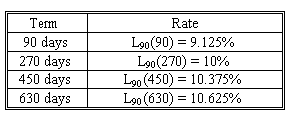What's the value of the swap?The value of the fixed payments, including the hypothetical notional principal, is:
VFXRB = (180/360) x 0.0975 x (0.9777 + 0.9302 + 0.8852 + 0.8432) + 1 x 0.8432 = 1.02046963.

The value of the floating payments, including the hypothetical notional principal, is based on discounting the next floating payment of 0.045 and the market value of the floating-rate bond on the next payment date, which is 1: VFLRB = (0.045 + 1) x (0.9777) = 1.0216965.

Thus, the value of the swap per \$1 notional principal is: VS = 1.0216965 - 1.02046963 = 0.00122687.

Taking into account the \$20 million notional principal, the value of the swap is \$20,000,000 x 0.00122687 = \$24,537.

To the counterparty, the value of the swap is -\$24,537.

#### Practice Question 3

Which of the following strategies is (are) appropriate?

I. If a borrower has a fixed rate debt and is expecting interest rates to rise, then the borrower should not enter into a swap.
II. If an investor has floating rate assets and is expecting interest rates to rise, then the investor should enter into a swap in order too receive fixed and pay float.
III. If a borrower has floating rate debt and is expecting interest rates to rise, then the borrower should enter into a swap in order to receive float and pay fixed.
IV. If an investor has fixed income assets and is expecting interest rates to drop, then the investor should enter into a swap in order to receive float and pay fixed.

II is incorrect because if an investor has floating rate assets and is expecting interest rates to rise, then the investor should not enter into a swap. If the asset has a floating rate, then as interest rates rise, so will the income from the asset.

IV is incorrect because if an investor has fixed income assets and is expecting interest rates to drop, then their assets will appreciate in value. Consequently, they should not hedge against this potential by entering into a swap.

#### Practice Question 4

Consider a one-year swap with quarterly payments on days 90, 180, 270 and 360. The underlying is 90-day LIBOR. The annualized LIBOR spot rates today are: L0(90) = 0.0345, L0(180) = 0.0358, L0(270) = 0.0370, L0(360) = 0.0375.

What should be the fixed rate of this swap?

A. 3.62%.
B. 3.68%.
C. 3.54%.

The present value factors are obtained as follows:

B0(90) = 1 / (1 + 0.0345 x 90/360) = 0.9914.
B0(180) = 1 / (1 + 0.0358 x 180/360) = 0.9824.
B0(270) = 1 / (1 + 0.0370 x 270/360) = 0.9730.
B0(360) = 1 / (1 + 0.0375 x 360/360) = 0.9639.
q = 90/360 = 0.25. R = 1/0.25 x (1 - 0.9639) / (0.9914 + 0.9824 + 0.9730 + 0.9639) = 0.0368, or 3.68%.

#### Practice Question 5

Suppose we have entered into a one-year swap with quarterly payments on days 90, 180, 270 and 360. The fixed rate we pay is 3.68% (annualized). The underlying is 90-day LIBOR. The notional principal is \$1,000,000. On day 0 the 90-day LIBOR was 3.45%. Suppose we have now moved 60 days into the life of the swap. At day 60, we face a new term structure of LIBORs which is given as follows:

L60(30) = 0.0425, L60(120) = 0.0432, L60(210) = 0.0437, L60(300) = 0.0444.

What is the value of the swap?

A. \$7,900.
B. \$5,300.
C. \$4,700.

The new set of discount factors is:
B60(90) = 1 / (1 + 0.0425 x 30/360) = 0.9965.
B60(180) = 1 / (1 + 0.0432 x 120/360) = 0.9858.
B60(270) = 1 / (1 + 0.0437 x 210/360) = 0.9751.
B60(360) = 1 / (1 + 0.0444 x 300/360) = 0.9643.

The present value of the remaining fixed payments of 0.0092 (0.0368/4), including the hypothetical notional principal, is 0.0092 x (0.9965 + 0.9858 + 0.9751 + 0.9643) + 1 x 0.9643 = 1.0004.

As the market value of the remaining payments on day 90, including the hypothetical final notional principal, is 1.0, we discount 1.00 + 0.0086 (which is 0.0345/4) = 1.0086 back 30 days to obtain 1.0086 x 0.9965 = 1.0051.

Therefore, the value of the swap is (1.0051 - 1.0004) x 1,000,000 = \$4,700.

#### Practice Question 6

Consider a one-year interest rate swap with semi-annual payments. The term structure of LIBOR spot rates is given as follows: L0(180) = 7.2%, and L0(360) = 8.0%. What is the annualized fixed rate on the swap?

A. 7.56%.
B. 7.84%.
C. 7.42%.

First calculate the present value factors for 180 and 360 days:
B0(180) = 1 / (1 + 0.072 x 180/360) = 0.9653.
B0(360) = 1 / (1 + 0.08 x 360/360) = 0.9259.

The fixed rate is (1/0.5) x (1 - 0.9259) / (0.9653 + 0.9259) = 0.0784, or 7.84%.

#### Practice Question 7

Consider a one-year interest rate swap with semi-annual payments. The annualized fixed rate is 7.84%. The term structure of LIBOR spot rates is given as follows:
On day 0: L0(180) = 7.2%, and L0(360) = 8.0%.
On day 90: L90(90) = 7.1%, and L90(270) = 7.4%.

Assume a notional principal of \$1 million. What is the market value of the swap from the perspective of the party paying the floating rate on day 90?

A. \$5,100.
B. \$4,200.
C. -\$4,200.

Calculate the new present value factors for 90 and 270 days:
B90(180) = 1 / (1 + 0.071 x 90/360) = 0.9826.
B90(360) = 1 / (1 + 0.074 x 270/360) = 0.9474.

The present value of the remaining fixed payments of 0.0392 (0.0784/2), including the hypothetical notional principal, is 0.0392 x (0.9826 + 0.9474) + 1 x 0.9474 = 1.0231.

As the market value of the remaining payments on day 90, including the hypothetical final notional principal, is 1.0, we discount 1.00 + 0.036 (which is 0.072/2) = 1.036 back 90 days to obtain 1.036 x 0.9826 = 1.0180.

Therefore, the value of the swap is (1.0231 - 1.018) x 1,000,000 = \$5,100.

#### Practice Question 8

Two parties enter into a three-year interest rate swap, which involves the exchange of LIBOR+1% for a fixed rate of 12% on a \$100 million notional amount. The LIBOR rate today is 11%, but is expected to increase to 15% in one year and fall back down to 8%. Which statement accurately depicts the flow of net cash flows between the two counter-parties?

A. The variable rate payer would receive a payment of \$4 million at the end of year two, while the fixed rate payer would receive \$3 million at the end of year three.
B. The fixed rate payer would receive a payment of \$4 million at the end of year two, while the variable rate payer would receive \$3 million at the end of year three.
C. The fixed rate payer will have to pay \$3 million at the end of the second year and \$3 million at the end of the third year.